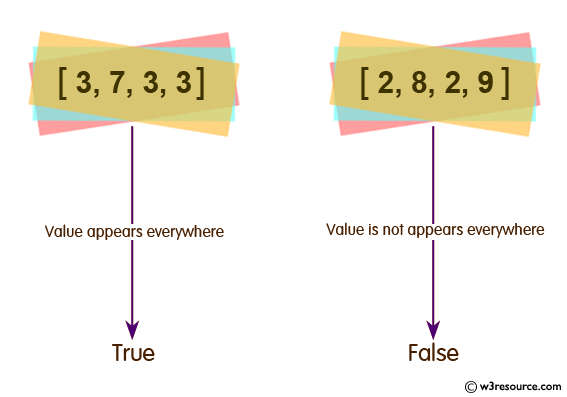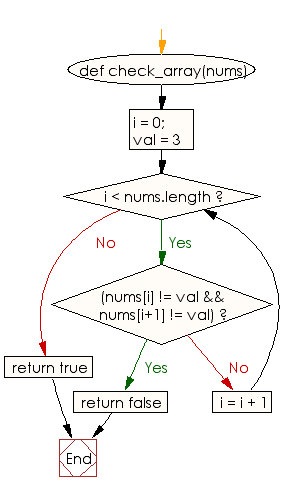﻿ Ruby Array exercises: Check whether a given value appears everywhere in an given array - w3resource# Ruby Array Exercises: Check whether a given value appears everywhere in a given array

## Ruby Array: Exercise-37 with Solution

Write a Ruby program to check whether a given value appears everywhere in an given array. A value is "everywhere" in an array if it presents for every pair of adjacent elements in the array.Ruby Code:

``````def check_array(nums)
i = 0;
val = 3
while i < nums.length
if(nums[i] != val && nums[i+1] != val)
return false
end
i = i + 1
end
return true
end
print check_array([3, 7, 3, 3]),"\n"
print check_array([2, 8, 2, 9]),"\n"
print check_array([3, 8, 5, 4, 3, 7]),"\n"
``````

Output:

```true
false
false
```

Flowchart:Ruby Code Editor: